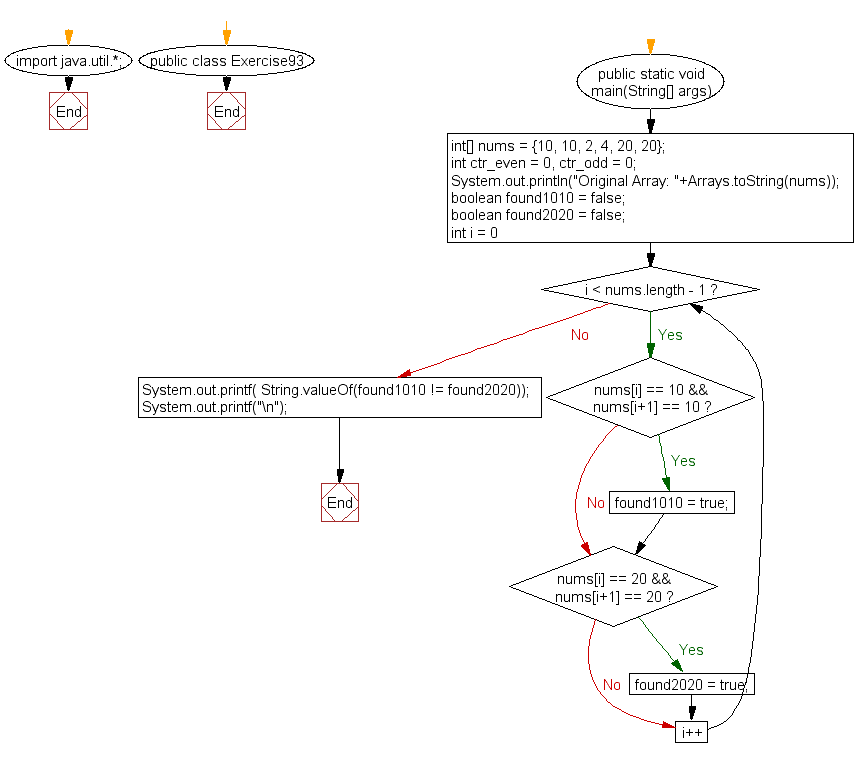﻿ Java: Test if an array contains an element 10 next to 10# Java Exercises: Test if an array of integers contains an element 10 next to 10 or an element 20 next to 20, but not both

## Java Basic: Exercise-93 with Solution

Write a Java program to test if an array of integers contains an element 10 next to 10 or an element 20 next to 20, but not both.

Sample Solution:

Java Code:

``````import java.util.*;
public class Exercise93 {
public static void main(String[] args)
{
//int[] nums = {10, 10, 2, 4, 9};
int[] nums = {10, 10, 2, 4, 20, 20};
int ctr_even = 0, ctr_odd = 0;
System.out.println("Original Array: "+Arrays.toString(nums));

boolean found1010 = false;
boolean found2020 = false;

for(int i = 0; i < nums.length - 1; i++) {
if(nums[i] == 10 && nums[i+1] == 10)
found1010 = true;

if(nums[i] == 20 && nums[i+1] == 20)
found2020 = true;
}

System.out.printf( String.valueOf(found1010 != found2020));
System.out.printf("\n");
}
}
```
```

Sample Output:

```Original Array: [10, 10, 2, 4, 20, 20]
false
```

Pictorial Presentation:Flowchart:Java Code Editor:

What is the difficulty level of this exercise?

Test your Programming skills with w3resource's quiz.

﻿

## Java: Tips of the Day

getEnumMap

Converts to enum to Map where key is the name and value is Enum itself.

```public static <E extends Enum<E>> Map<String, E> getEnumMap(final Class<E> enumClass) {
return Arrays.stream(enumClass.getEnumConstants())
.collect(Collectors.toMap(Enum::name, Function.identity()));
}
```

Ref: https://bit.ly/3xXcFZt